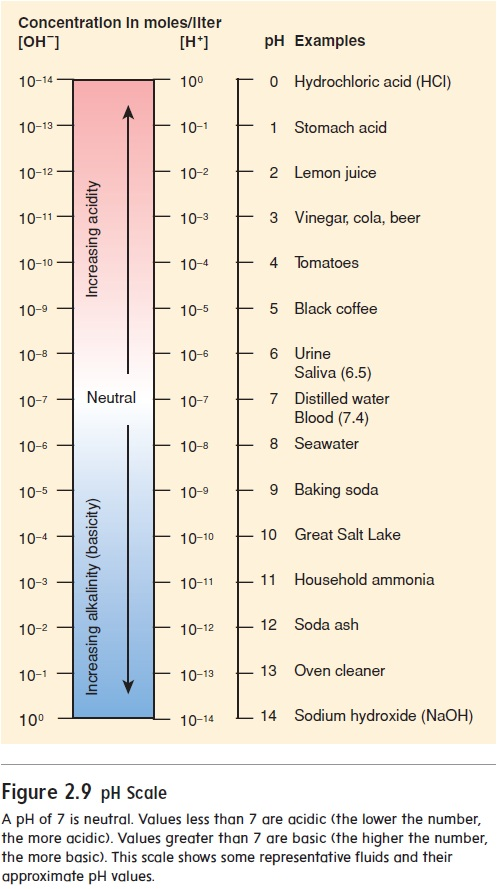# Acids and BasesA.Describe the pH scale and its relationship to acidic andbasic solutions. B. Explain the importance of buffers in organisms.

ACIDS AND BASICS

A.Describe the pH scale and its relationship to acidic and basic solutions.

B. Explain the importance of buffers in organisms.

The body has many molecules and compounds, called acids and bases, that can alter body functions. An acid is a proton donor. Because a hydrogen atom without its electron is a proton, any substance that releases hydrogen ions (H+) in water is an acid. For example, hydrochloric acid (HCl) in the stomach forms H+ and chloride ions (Cl):

HCl  H+ + Cl

base is a proton acceptor. For example, sodium hydroxide (NaOH) forms sodium ions (Na+) and hydroxide ions (OH). It is a base because the OH is a proton acceptor that binds with a H+ to form water.## The pH Scale

The pH scale indicates the H+ concentration of a solution (figure 2.9). The scale ranges from 0 to 14. A neutral solution has an equal number of H+ and OH and thus a pH of 7.0. An acidic solution has a greater concentration of H+ than of OH and thus a pH lessthan 7.0. A basic, or alkaline (al′\kă-lı̄n), solution has fewer H+ than OH and thus a pH greater than 7.0. Notice that the pH num-ber and the actual H+ concentration are inversely related, meaning that the lower the pH number, the higher the H+ concentration.As the pH value becomes smaller, the solution becomes more acidic; as the pH value becomes larger, the solution becomes more basic. A change of one unit on the pH scale represents a 10-fold change in the H+ concentration. For example, a solution with a pH of 6.0 has 10 times more H+ than a solution with a pH of 7.0. Thus, small changes in pH represent large changes in H+concentration.

The normal pH range for human blood is 7.35 to 7.45. If blood pH drops below 7.35, a condition called acidosis (as-i-dō′\sis) results. The nervous system is depressed, and the individual becomes disoriented and possibly comatose. If blood pH rises above 7.45, alkalosis (al-kă-lō′\sis) results. The nervous system becomes overexcitable, and the individual can be extremely nervous or have convulsions. Both acidosis and alkalosis can result in death.

A pH of 7 is neutral. Values less than 7 are acidic (the lower the number, the more acidic). Values greater than 7 are basic (the higher the number, the more basic). This scale shows some representative fluids and their approximate pH values.

## Salts

salt is a compound consisting of a positive ion other than H+ and a negative ion other than OH. Salts are formed by the reac-tion of an acid and a base. For example, hydrochloric acid (HCl) combines with sodium hydroxide (NaOH) to form the salt sodium chloride (NaCl):## Buffers

The chemical behavior of many molecules changes as the pH of the solution in which they are dissolved changes. The survival of an organism depends on its ability to maintain homeostasis by keeping body fluid pH within a narrow range. One way normal body fluid pH is maintained is through the use of buffers. A buffer (bŭf′\er) is a chemical that resists changes in pH when either an acid or a base is added to a solution containing the buffer. When an acid is added to a buffered solution, the buffer binds to the H+, preventing these ions from causing a decrease in the pH of the solution (figure 2.10).Study Material, Lecturing Notes, Assignment, Reference, Wiki description explanation, brief detail
Essentials of Anatomy and Physiology: The chemical basis of life : Acids and Bases |

Related Topics